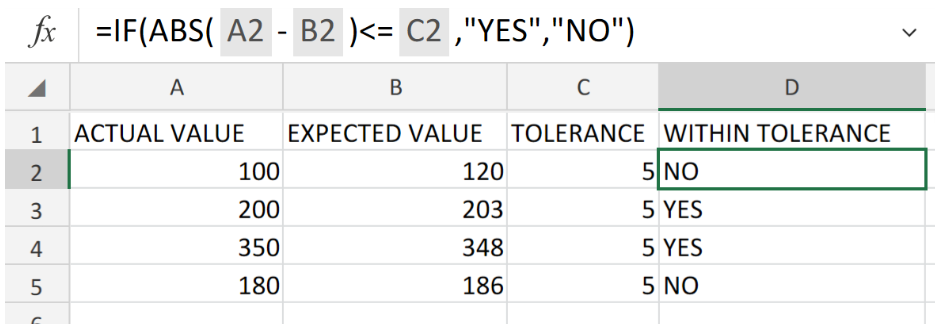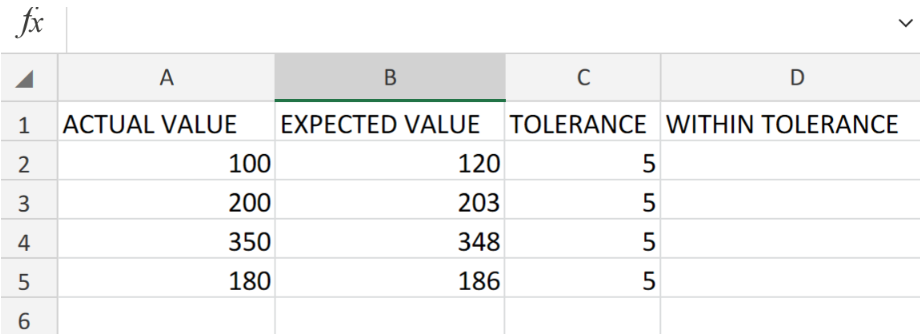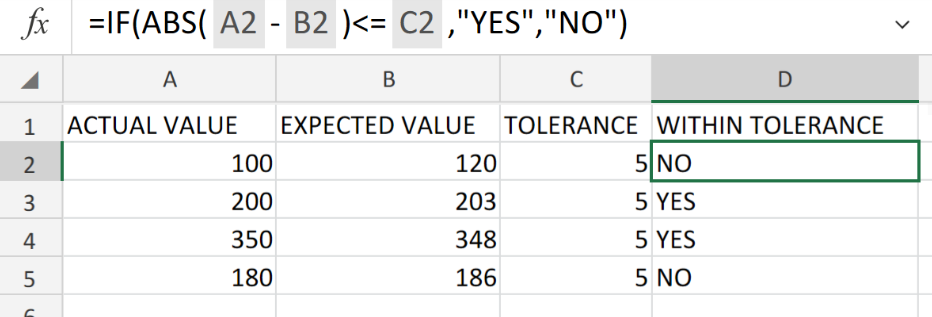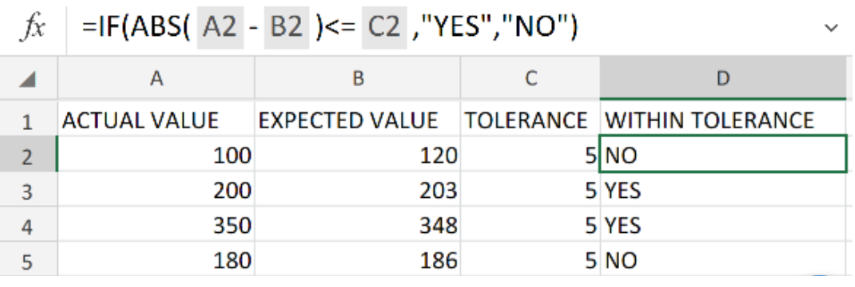Get instant live expert help with Excel or Google Sheets“My Excelchat expert helped me in less than 20 minutes, saving me what would have been 5 hours of work!”

#### Post your problem and you’ll get expert help in seconds.

Your message must be at least 40 characters
Our professional experts are available now. Your privacy is guaranteed.

# Determine If a Value Is Within a Tolerance in ExcelFigure 1. of Value Within Tolerance in Excel.

In order to determine whether or not a value is within our expected level of tolerance, we are going to utilize the ABS and IF Functions in Excel. This article will walk through the process

## Generic Formula

`=IF(ABS(actual-expected)<=tolerance,”Yes","No")`

The Excel “IF” function calculates whether or not a value logically or mathematically satisfies a set of specified conditions.

## How to use the IF and ABS Functions in Excel.

We are going to test whether or not some data values fall within our expected tolerance levels by following 3 simple steps;

1. Label the ACTUAL VALUE,  EXPECTED VALUE and TOLERANCE columns in our worksheet.

Also, have the results column (WITHIN TOLERANCE) ready as shown in the example illustrated below;Figure 2. of Value Within Tolerance Table in Excel.

1. Now, in the event that we want to discover if the expected values (in column B above),  fall within our budget tolerance levels (in column C above), we are going to enter the following IF and ABS operation syntax into cell D2 of our worksheet;

`=IF(ABS(A2-B2)<=C1:C2,"YES","NO")`Figure 3. of the IF and ABS Operation Syntax in Excel.

1. Copy the IF and ABS operation syntax into into the cells down the entire column D to achieve the desired results for each value.

In the example, our Expected data value is subtracted from the Actual or Measured data value. Our resulting value may be negative or positive, depending on our Actual data value. Thus, we can utilize the Excel ABS Function in order to convert all of our results into positive values.

The formula then proceeds to compare this positive result to our specified tolerance level, by way of a logical test within the IF formula syntax. In the event that the difference is less than or equal to our specified tolerance level, IF is returned as “YES”. In the event that the difference is greater than or equal to our specified tolerance level, IF is returned as “NO”.Figure 4. of Final Result.

## Instant Connection to an Expert through our Excelchat Service

Our live Excelchat Service is here for you. We have Excel Experts available 24/7 to answer any Excel questions you may have. Guaranteed connection within 30 seconds and a customized solution for you within 20 minutes.

### Did this post not answer your question? Get a solution from connecting with the expert.Another blog reader asked this question today on Excelchat:
Related blogs
Solution examplesGiven: RetYr = 2057 B48=2055 B49=2056 B50=2057 FirstYrAnnExp = 174,855 B51=2058 M48=IF(B48>=RetYr-1,IF(M47>1,M47*(1+Inflation),FirstYrAnnExp)) Returns FALSE M49=IF(B49>=RetYr-1,IF(M48>1,M48*(1+Inflation),FirstYrAnnExp)) Correctly retuns 0 M50=IF(B50>=RetYr-1,IF(M49>1,M49*(1+Inflation),FirstYrAnnExp)) Correctly returns 174,855 M51=IF(B51>=RetYr-1,IF(M50>1,M50*(1+Inflation),FirstYrAnnExp)) Correctly returns 180,100 How can I avoid â€œFALSEâ€ when the B column is less than RetYr? A zero or blank would be better.
Solved by V. W. in 20 minsusing excel as a checkbook -- formula =IF(ISBLANK(E283),"",N282-J283+L283) after 280 lines it is now just saying VALUE in the balance column (N) What have I done wrong?
Solved by T. Y. in 44 minsI want a formula which says if x =" y" or "z" or "whatever" multiple a by either "x" or "y" but get a VALUE error- any ideas? =PRODUCT(IF('Historical data'!I5="Research Associate",Costs!\$B\$18*'Historical data'!F5),PRODUCT(IF('Historical data'!I5="Senior Research Associate A",Costs!\$C\$18*'Historical data'!F5),PRODUCT(IF('Historical data'!I5="Senior Research Associate B",Costs!\$D\$18*'Historical data'!F5),PRODUCT(IF('Historical data'!I5="Freelancers",Costs!\$E\$18*'Historical data'!F5),PRODUCT(IF('Historical data'!I5="Research Director",Costs!\$F\$18*'Historical data'!F5),PRODUCT(IF('Historical data'!I5="Company Director",Costs!\$G\$18*'Historical data'!F5),PRODUCT(IF('Historical data'!I5="Managing Director",Costs!\$H\$18*'Historical data'!F5),"")))))))
Solved by V. Y. in 20 minshello i need help creating a formula, if a value does not exsits put 0, if a value exist do nothing
Solved by M. F. in 9 minsCompute the weighted average of students score from a spreadsheet using different weight for each category of marks
Solved by G. E. in 20 mins## Subscribe to Excelchat.coAnother blog reader asked this question today on Excelchat: